Next: The fast Fourier transform Up: Poisson's equation Previous: 2-d problem with Dirichlet

## 2-d problem with Neumann boundary conditions

Let us redo the above calculation, replacing the Dirichlet boundary conditions (149) with the following simple Neumann boundary conditions: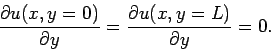(161)

In this case, we can express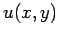in the form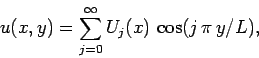(162)

which automatically satisfies the boundary conditions in the-direction. Likewise, we can write the source term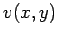as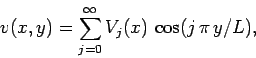(163)

where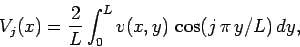(164)

since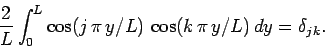(165)

Finally, the boundary conditions in the-direction become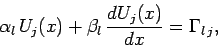(166)

at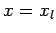, and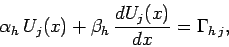(167)

at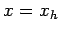, where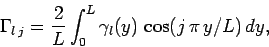(168)

etc. Note, however, that the factor in front of the integrals in Eqs. (164) and (168) takes the special value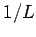for the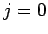harmonic.

As before, we truncate the Fourier expansion in the-direction, and discretize in the-direction, to obtain the set of tridiagonal matrix equations specified in Eqs. (158), (159), and (160). We can solve these equations to obtain the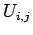, and then reconstruct the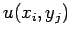from Eq. (162). Hence, we have solved the problem.Next: The fast Fourier transform Up: Poisson's equation Previous: 2-d problem with Dirichlet
Richard Fitzpatrick 2006-03-29